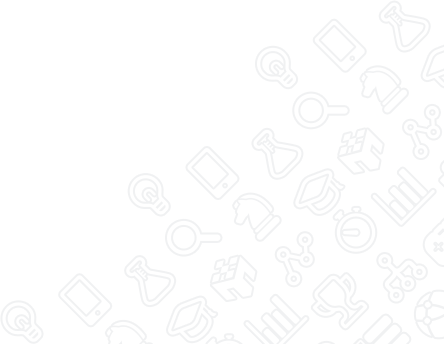MobLab
Instructions
Aa

# Double Marginalization: No Integration

## How to Play

• Wholesalers: Use +/- buttons to alter the price per bag of coffee charged to the retailer then press Make Offer to post a price.
• Retailers: After the wholesaler sets a price you know your cost per bag. Use your +/- to buttons to determine how much quantity to order from them at that price. Then, press the Place Order button.
• For the retailer the market price for bags of coffee is determined by their quantity choice.
• All: Once the wholesaler sets a price and the retailer chooses quantity both will receive their profit information.

## Payoff Computation

• Wholesalers: A wholesaler’s per bag profit is the price charged to a retailer minus the cost of production \$r - \$MC. Their total profit can be found by multiplying per bag profit by the total number of bags sold: Qx(\$r - \$MC)
• Retailers: A retailer’s per bag profit is the price charged to the customer minus the price paid to the wholesaler \$P - \$r. Their total profit can be found by multiplying per bag profit by the total number of bags sold: Qx(\$P - \$r)

## Example

#### Example 1

The inverse market demand for bags of coffee is given by P = 12 - Q. The marginal cost to the wholesaler for coffee production is MC=\$4.

Wholesaler sets a price of r=\$7. The retailer then chooses Q. Given a market demand curve of P = 12 - Q if the retailer chooses Q=4 that means the market price is P=\$8.

So ultimately profits are:

Retailer: Qx(\$P - \$r) = 4x(\$8 - \$7) = \$4
Wholesaler: Qx(\$r - \$MC) = 4x(\$7-\$4) = \$12

#### Example 2

We use the same inverse demand curve P = 12 - Q and marginal cost MC=\$4.

Suppose the wholesaler sets a price of r=\$5. Then, the retailer chooses Q=6 units at r=\$5. Given a market demand curve of P = 12 - Q if the retailer chooses Q=6 that means the market price is P=\$6.

So ultimately profits are:

Retailer: Qx(\$P - \$r) = 6x(\$6 - \$5) = \$6
Wholesaler: Qx(\$r - \$MC) = 6x(\$5-\$4) = \$6